# MROUND Function

Returns a number rounded to a specified multiple

## What is the MROUND Function?

The MROUND function is categorized under Excel Math and Trigonometry functions. The function will return a number rounded to a multiple given by the user. MROUND will always round up away from zero.

As a financial analyst, the MROUND function helps round a number and eliminate the least significant digits, making it simpler but keeping close to the original value.

### Formula

=MROUND(number, multiple)

The MROUND function uses the following arguments:

1. Number (required argument) – This is the number that needs to be rounded.
2. Multiple (required argument) – This is the multiple to which we want to round a number.

### How to use the MROUND Function in Excel?

To understand the uses of the MROUND function, let’s consider a few examples:

#### Example 1

Suppose we are given the following data: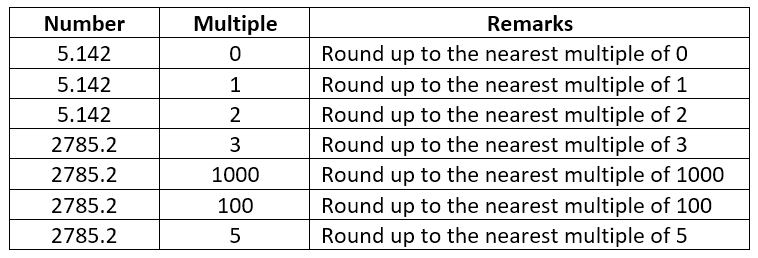The results in MS Excel are shown below: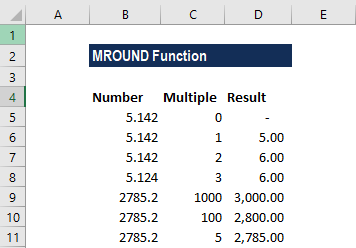#### Example 2

Let’s see how the MROUND function can be used to get a time rounded up to the nearest 30-minute interval. Suppose we are given the data below: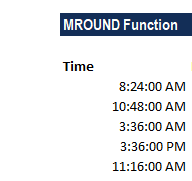The formula used is shown below: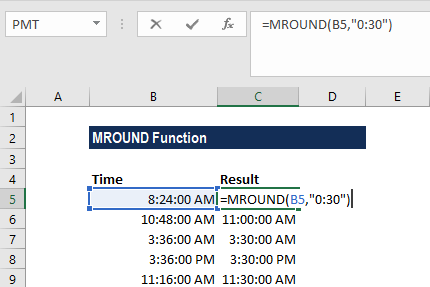We get the result below: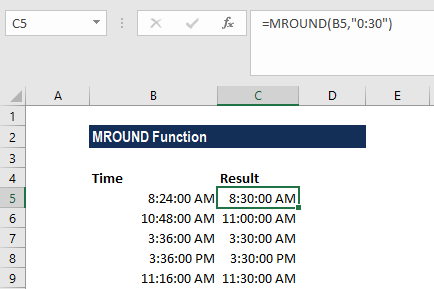When we supply “0:30” as the multiple, Excel will internally convert 0:30 into 0.0208333333333333, which is the decimal value that represents 30 minutes.

We can also express 30 minutes in a formula using the formula =30/(60*24).

Alternatively, depending on the requirements, we can use the formula to round to different time intervals such as the nearest 15 minutes or 1 hour.

### Few notes about the MROUND Function

1. MROUND rounds up away from zero.
2. The number and multiple arguments must be of the same sign.
3. #NUM! error – Occurs when the number and multiple arguments are not of the same sign.

Thanks for reading CFI’s guide to this important Excel function. By taking the time to learn and master these Excel functions, you’ll significantly speed up your financial analysis. To learn more, check out these additional CFI resources:

• Excel Functions for Finance